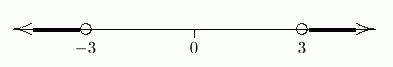#Solving Simple Absolute Value Sentences

Need some simpler practice with absolute value first?

Recall that $\,|x|\,$ gives the distance between $\,x\,$ and $\,0\,.$ If you think in terms of distance, then it's easy to solve sentences involving absolute value!

## Example: An Absolute Value Equation

Solve: $|x| = 3$
Answer: $x = 3\ \text{ or }\ x=-3$

Note: We want all numbers $\,x\,$ whose distance from zero is $\,3\,.$

$$\cssId{s16}{\overset{\text{whose distance from zero ...}}{\overbrace{\ |\overset{\text{want numbers \,x\,... }}{\ \strut x\ }|\ }} \overset{\text{is ...}}{\strut \ \ \ =\ \ \ } \overset{\text{three}}{\strut 3}}$$

The diagram above shows how the sentence is telling you what you want! Interpret it in the following order:

 1) We want numbers $\,x\,$... the unknown is $\,x\,$ 2) whose distance from zero ... the vertical bars, $|\ |\,,$ ask for distance from zero 3) is ... the equal sign 4) $3$ three

Remember that you can ‘walk’ from zero in two directions: to the right, and to the left. The number $\,3\,$ is three units from zero to the right; the number $\,-3\,$ is three units from zero to the left.The word ‘or’ in the sentence ‘$\,x=3\text{ or }x=-3\,$’ is the mathematical word ‘or’. You may want to review its meaning:

## Example: An Absolute Value Inequality Involving ‘Less Than’

Solve: $|x| \lt 3$
Answer: $-3 \lt x \lt 3$

Note: We want all numbers $\,x\,$ whose distance from zero is less than $\,3\,.$

$$\cssId{s40}{\overset{\text{whose distance from zero ...}}{\overbrace{\ |\overset{\text{want numbers \,x\,... }}{\ \strut x\ }|\ }} \overset{\text{is less than ...}}{\strut \ \ \ \ \ \lt\ \ \ \ \ } \overset{\text{three}}{\ \ \ \ \strut 3\ \ \ \ }}$$

The diagram above shows how the sentence is telling you what you want! Interpret it in the following order:

 1) We want numbers $\,x\,$... the unknown is $\,x\,$ 2) whose distance from zero ... the vertical bars, $|\ |$, ask for distance from zero 3) is less than ... the ‘less than’ symbol 4) $3$ three

You can walk less than three units to the right—this gets you the numbers from $\,0\,$ to $\,3\,.$ You can walk less than three units to the left—this gets you the numbers from $\,0\,$ to $\,-3\,.$ Together, you end up with all the numbers between $\,-3\,$ and $\,3\,$:The sentence ‘$\,-3 \lt x \lt 3\,$’ is just a shorthand for ‘$\,-3\lt x\,$ and $\,\ x \lt 3\,$’. That's the mathematical word ‘and’. You may want to review its meaning:

The shorthand ‘$\,-3\lt x\lt 3\,$’ is a great shorthand, because you see an $\,x\,$ trapped between $\,-3\,$ and $\,3\,$; and those are precisely the values of $\,x\,$ that make the sentence true.

## Example: An Absolute Value Inequality Involving ‘Greater Than’

Solve: $|x| \gt 3$
Answer: $x \lt -3\ \text{ or }\ x\gt 3$

Note: We want all numbers $\,x\,$ whose distance from zero is greater than $\,3\,.$

$$\cssId{s66}{\overset{\text{whose distance from zero ...}}{\overbrace{\ |\overset{\text{want numbers \,x\,... }}{\ \strut x\ }|\ }} \overset{\text{is greater than ...}}{\strut \ \ \ \ \ \gt\ \ \ \ \ } \overset{\text{three}}{\ \ \ \ \strut 3\ \ \ \ }}$$

The diagram above shows how the sentence is telling you what you want! Interpret it in the following order:

 1) We want numbers $\,x\,$... the unknown is $\,x\,$ 2) whose distance from zero ... the vertical bars, $|\ |$, ask for distance from zero 3) is greater than ... the ‘greater than’ symbol 4) $3$ three

You can walk more than three units to the right—this gets you all the numbers to the right of $\,3\,.$ You can walk more than three units to the left—this gets you all the numbers to the left of $\,-3\,.$ Together, you end up with the two pieces shown below:The word ‘or’ in the sentence ‘$\,x\lt -3\,$ or $\, x\gt 3\,$’ is the mathematical word ‘or’. You may want to review its meaning:

## Example: An Absolute Value Sentence With No Solutions

Solve: $|x| \lt -1$
Solution: There are no solutions, since distance can't be negative. That is, $\,|x|\,$ is always greater than or equal to zero; so, it can't ever be less than $\,-1\,.$

## Example: An Absolute Value Sentence That Is Always True

Solve: $|x| \gt -1$
Solution: All real numbers are solutions, since all distances are nonnegative. That is, $\,|x|\,$ is always greater than or equal to zero; so, it must also be greater than $\,-1\,.$

## Concept Practice

Solve the given absolute value sentence. Write the result in the most conventional way.

For more advanced students, a graph is available. For example, the equation $\,|x| = 3\,$ is optionally accompanied by the graph of $\,y = |x|\,$ (the left side of the equation, dashed green) and the graph of $\,y = 3\,$ (the right side of the equation, solid purple). In this example, you are finding the values of $\,x\,$ where the green graph intersects the purple graph.

Click the ‘Show/Hide Graph’ button to toggle the graph.

Solve: